﻿ Math and Date Math

# Math and Date Math Features (Instant Database)

Simple math can be performed using any existing Instant Database variable which has been assigned a numeric value. Create a formulas and assign its result to another variables.

Pathagoras can perform addition, subtraction, multiplication or division on up to four operands.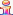This feature presupposes that you will be preparing an Instant Database mask to house the formulas. If you want to place the formula in-line with the text of the source document, see this page.

Example: Let's say that you have a document containing, among others, four variables that you want to use to  calculate a total price. Perhaps they are called [Unit Price], [Quantity], [Discount] and [Total Price]. These variables are contained in a mask designed for this and similar 'price quoting' documents.

The formula in the mask adjacent to [Total Price] might look like one of these:

[Unit Price] * [Quantity] - [Discount] (if the variable discount is a flat amount) OR

[Unit Price] * [Quantity] * [Discount] (if the variable 'discount' is a percentage) OR

[Unit Price] *  * .25 (you can use 'real' numbers if you wish, with or without brackets).

[Unit Price] * 150 * .25

In actual operation, you would assemble the document for the customer and display the appropriate IDB mask. You would complete the 'personal variables' (name, address, etc.) and insert the appropriate values for each of the first three variables (unit price, quantity and discount) which feed the formula.

When Pathagoras detects a formula (the presence of math symbols is the give away) in the personal data side of the IDB screen, it will display a small red button between the two columns. (Similar in size to the ‘date picker’ button.) Click the red button and the formula is processed.

If any figure that is part of the formula changes, the [Total Price] can be recalculated by two successive clicks of the calculator button. The first click restores the formula. The second click recalculates using the new values.

You can have a total of 4 "operands" (and therefore up to 3 operators).

Here is another example: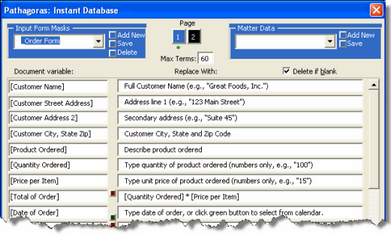A mask called Order Form was created for quoting prices. It contains a simple formula to calculate the value for
the variable called [Total of Order].When the mask is completed, and after values have been assigned to the base variables upon which the formula depends, click the red Calculate button. If a base figure changes, click the button twice to recalculate the value.

The allowable 'math operators' are:

Subtraction: "-"

Multiplication: "*" or "x"

Division: "/"

 In the examples on this page, you will see an introductory '=' sign in some and not in others. When all variables appear above the formula, the '=' is not required. The better practice would be to include the '=' in all cases.

DATE MATH:

Date math (addition) can be performed from the Instant Database screen following the same setup styles as used with the above 'plain' math examples. The formula is written like this:

=[Base Date]+[4 months]

where [Base Date] is any date variable in the mask (the variable must contain the word 'date', but it can be in any form). The time interval addend can be any number, followed by a space, and concluded with either 'days', 'weeks', 'months' or 'years' (or the singular).

Example:(1). Set the 'base' date by clicking the green 'Calendar' button. (You can also manually type in the date).

(2). Click the red 'Calculate' button on the target line. You should see the result as shown below: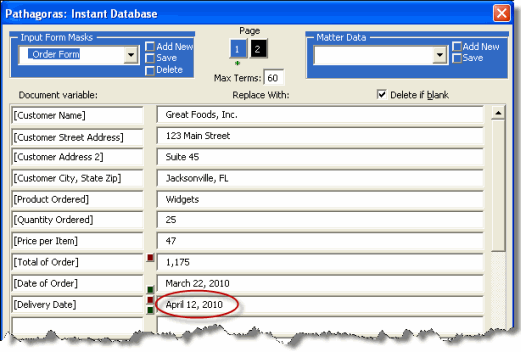If you change the 'base date,' click the calculate button twice.
The first click will restore the formula (in case you want to modify it).
The second click will perform the calculation on the new assumptions.

Date "difference" math (how many days, weeks months or years have passed between two known dates) can be performed from the Instant Database screen, again using a mask to save the formulas for repeated use.

The same setup styles as shown above is used.  Sample variables, a sample formula and screen shots, are provided below:

[Base date]

[End date]

[Span, in Months] =[Begin Date]-[End date] (the formula beginning with '=' is placed in the right column)

[Age in Years] =[Begin Date]-[End date]   (the formula beginning with '=' is placed in the right column)

Other acceptable calls:

[Age in Years and Months] will return e.g., 10 years and 9 months
or
[Age in YM] (same as above)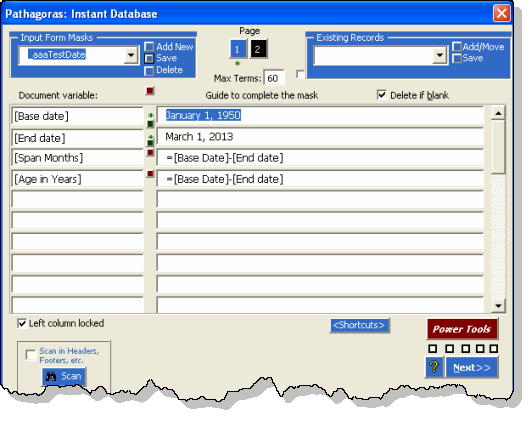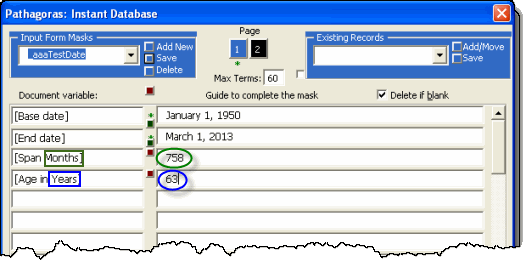There are 758 months between the two dates, which converts to 63 (whole) years.
The numeric result of number of days, weeks, months or years is controlled by the existence of
the appropriate term "day(s)", "week(s)", "month(s)" or "year(s)"
contained in the variable at the left.

NOTES and SETUP REQUIREMENTS:

As the above examples suggest, the operands used in a Date Math formula must be set ‘above’ the location where the math is being performed. Pathagoras cannot look ‘down’ for a value. This is not an issue when operand variables all appear in the document before the math equation. But when they do not, you can 'force' the apppearance of the necessary varible(s) to be above the math equation in two ways:

Inconspicuously (maybe in an information collection section) add the required variable above the math equation so Pathagoras picks it up first in its top-to-bottom document scan.

Create an Input Form Mask with the rearranged variables and always us the Mask (instead of scanning) to insure the correct order of the variables.